# Solid

In the context of Geometry, a solid is a shape that occupies space and has volume and surface area. A solid has three dimensions, including length, width (or depth), and height. The following are some examples.

## Types of solids

While there are many different ways to categorize solids, solids are often divided into polyhedra (plural for polyhedron) and non-polyhedra. The following are some important polyhedra and non-polyhedra studied in Geometry.

### Polyhedron

A polyhedron is a solid whose faces are polygons. The word polyhedron has Greek origins, which loosely translates as "many faces." Prisms and pyramids are two of many types of polyhedra studied in geometry.

#### Prism

Prisms are polyhedra that have two congruent faces, called bases, that lie in parallel planes. The other faces (the sides that are not bases) are parallelograms, rectangles, or squares. A prism is typically named based on the shape of its polygonal bases. Below are three examples.

Rectangular prism Hexagonal prism Triangular prism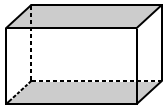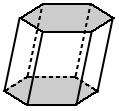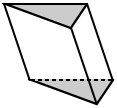The bases are rectangles. The bases are hexagons. The bases are triangles.

#### Pyramids

Pyramids are polyhedra that contain only one polygonal base; all the other faces are triangles. The triangular faces (aside from the base of a triangular pyramid) all share a common vertex called the apex. Like a prism, a pyramid is also typically named based on the shape of its polygonal base. Below are three examples of pyramids with different bases.

Square pyramid Triangular pyramid Hexagonal pyramid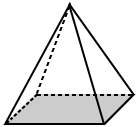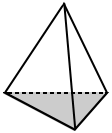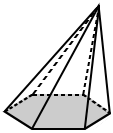The base is a square. The base is a triangle. The base is a hexagon.

#### General polyhedra

Polyhedra are 3D shapes that can take on many other shapes. Below are two examples.

### Non-polyhedra

A non-polyhedron is a space figure that has at least one face or component that is not a polygon. In geometry, three types of non-polyhedra that are commonly studied are cylinders, cones, and spheres.

#### Cylinder

A cylinder is a 3D shape that is made up of two flat circular bases and a curved surface that "wraps" around the bases. The following are two examples.

#### Cone

A cone is a 3D shape that is made up of a circular base that tapers to a point. The following are two examples.

#### Sphere

A sphere is a 3D shape that has a round shape. All points on the surface of a sphere are equidistant from a point called the center.